# MCQs on Electric Circuits

##### Page 31 of 63. Go to page 1 2 3 4 5 6 7 8 9 10 11 12 13 14 15 16 17 18 19 20 21 22 23 24 25 26 27 28 29 30 31 32 33 34 35 36 37 38 39 40 41 42 43 44 45 46 47 48 49 50 51 52 53 54 55 56 57 58 59 60 61 62 63
01․ A capacitance is a circuit component that opposes the change in circuit
voltage.
current.
impedance.
all of the above.

When there is an instantaneous reduction in the voltage, capacitors provide current to the voltage source, which partially compensate the original reduction in the voltage.

02․ The inverse of capacitance is called
reluctance.
conductance.
susceptance.
elastance.

The elastance creates the voltage across a capacitor after accepting an electric charge of 1 coulomb; it is the reciprocal of the farad. The unit of elastance is the Daraf, but is not recognized by SI.

03․ The sparking between two electrical contacts can be reduced by inserting a
capacitor in parallel with contacts.
capacitor in series with each contacts.
resistance in line.
none of above.

An arc between two electrodes can be initiated by ionization of atmospheric air. If we connect a capacitor across the contacts of the electrodes, there is a chance to make a short circuit by capacitor. Hence, there is no air gap between contacts and thereby sparking can be reduced.

04․ In a capacitive circuit, the capacitive reactance is
directly proportional to frequency
indirectly proportional to frequency
directly proportional to the square of frequency
indirectly proportional to the square of frequency

The capacitive reactance can be expressed as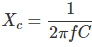05․ In a capacitor the electric charge is stored in
metal plates.
dielectric.
both of (A) and (B).
none of above.

In capacitors, the metal plates are used to connect a voltage source in between the dielectric medium. Hence, the electric charge is produced due to the presence of dielectric medium and it is stored in that dielectric medium itself.

06․ Three capacitors each of capacity C are given. The resultant capacity (2/3) C can obtained by using them
all in series.
all in parallel.
two in parallel and third in series with this combination.
two in series and third in parallel across this combination.

We know that the capacitance value for parallel connected capacitor is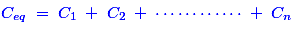and for series connected capacitors is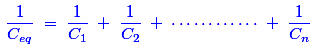Here, three capacitors have same value. Then, that the capacitance value for two parallel connected capacitor is ( C1+ C2 ) = (C + C ) = 2C. Now, this parallel connected combination is connected with one series connected capacitor. Hence, the final value of capacitance is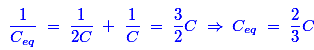07․ The capacitance of a capacitor is not affected by
distance between plates.
area of plates.
thickness of plate.
all of them.

The capacitance for any pair of separated conductors can be found with the below given expression: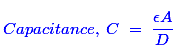Where, µ is Permittivity of dielectric, A is Area of the plates, D is Distance between the plates. So, the distance and area could affect the changes in the capacitance of a capacitor. Hence, thickness of plate does not affect the capacitance value.

08․ If the sheet of a backelite is inserted between the plates of an air capacitor, the capacitance will
decrease.
increase.
becomes zero.
remains unchanged.

Any electric charge produced by applied voltage and kept in bounds by an insulator (dielectric) that creates a dielectric field. Basically, Bakelite is a dielectric material (or) insulator and it has more dielectric strength than air medium. Due to the presence of this material in between the plates of an air capacitor, this can create the more dielectric field. Hence, the production of electric charge is high and thereby the capacitance value increases.

09․ A capacitor stores 0.24 coulombs at 10 volts. Its capacitance is
0.024 F.
0.12 F.
0.60 F.
0.80 F

We know that the capacitance is the ratio of charge and voltage. So,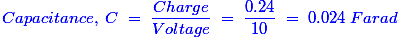10․ An electrolytic capacitor can be used for
DC only.
AC only.
both AC as well as AC.
none of above.

The electrolytic capacitors can be used in DC only because it is a polarized capacitor. There is a clear marking of the polarity (positive and negative) at the terminal point. We cannot used this capacitor in AC power supply because the electrolytic oxide material works in very low frequency power supply (nearly to be zero). If there is any incorrect polarization or presence of AC supply, the insulated oxide layer will break down very soon and it causes permanent damage as a result.

<<<2930313233>>>